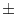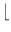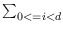Next: Real-Valued Vectors ( real_vector Up: Number Types and Linear Previous: Matrices with Integer Entries   Contents   Index

# Rational Vectors ( rat_vector )

Definition

An instance of data type rat_vector is a vector of rational numbers. A d-dimensional vector r = (r0,..., rd-1) is represented in homogeneous coordinates (h0,..., hd), where ri = hi/hd and the hi's are of type integer. We call the ri's the cartesian coordinates of the vector. The homogenizing coordinate hd is positive.

This data type is meant for use in computational geometry. It realizes free vectors as opposed to position vectors (type rat_point). The main difference between position vectors and free vectors is their behavior under affine transformations, e.g., free vectors are invariant under translations.

rat_vector is an item type.

#include < LEDA/numbers/rat_vector.h >

Creation

 rat_vector v(int d=2) introduces a variable v of type rat_vector initialized to the zero vector of dimension d. rat_vector v(integer a, integer b, integer D) introduces a variable v of type rat_vector initialized to the two-dimensional vector with homogeneous representation (a, b, D) if D is positive and representation (- a, - b, - D) if D is negative. Precondition D is non-zero. rat_vector v(rational x, rational y) introduces a variable v of type rat_vector initialized to the two-dimensional vector with homogeneous representation (a, b, D), where x = a/D and y = b/D. rat_vector v(integer a, integer b, integer c, integer D) introduces a variable v of type rat_vector initialized to the three-dimensional vector with homogeneous representation (a, b, c, D) if D is positive and representation (- a, - b, - c, - D) if D is negative. Precondition D is non-zero. rat_vector v(rational x, rational y, rational z) introduces a variable v of type rat_vector initialized to the three-dimensional vector with homogeneous representation (a, b, c, D), where x = a/D, y = b/D and z = c/D. rat_vector v(const array& A) introduces a variable v of type rat_vector initialized to the d-dimensional vector with homogeneous coordinates (c0,...,cd-1,D), where d = A.size() and A[i] = ci/D, for i = 0,..., d - 1. rat_vector v(integer a, integer b) introduces a variable v of type rat_vector initialized to the two-dimensional vector with homogeneous representation (a, b, 1). rat_vector v(const integer_vector& c, integer D) introduces a variable v of type rat_vector initialized to the vector with homogeneous coordinates (c0,...,cd-1,D), where d is the dimension of c and the sign chosen is the sign of D. Precondition D is non-zero. rat_vector v(const integer_vector& c) introduces a variable v of type rat_vector initialized to the direction with homogeneous coordinate vectorc, where the sign chosen is the sign of the last component of c. Precondition The last component of c is non-zero. rat_vector v(const vector& w, int prec) introduces a variable v of type rat_vector initialized to (P*w0,...,P*wd-1, P), where d is the dimension of w and P = 2prec.

Operations

3.1 Initialization, Access and Conversions

 rat_vector rat_vector::d2(integer a, integer b, integer D) returns a rat_vector of dimension 2 initialized to a vector with homogeneous representation (a, b, D) if D is positive and representation (- a, - b, - D) if D is negative. Precondition D is non-zero. rat_vector rat_vector::d3(integer a, integer b, integer c, integer D) returns a rat_vector of dimension 3 initialized to a vector with homogeneous representation (a, b, c, D) if D is positive and representation (- a, - b, - c, - D) if D is negative. Precondition D is non-zero. rat_vector rat_vector::unit(int i, int d=2) returns a rat_vector of dimension d initialized to the i-th unit vector. Precondition 0 < = i < d. rat_vector rat_vector::zero(int d=2) returns the zero vector in d-dimensional space. int v.dim() returns the dimension of v. integer v.hcoord(int i) returns the i-th homogeneous coordinate of v. rational v.coord(int i) returns the i-th cartesian coordinate of v. rational v[int i] returns the i-th cartesian coordinate of v. rational v.sqr_length() returns the square of the length of v. vector v.to_float() returns a floating point approximation of v.

Additional Operations for vectors in two and three-dimensional space

 rational v.xcoord() returns the zero-th cartesian coordinate of v. rational v.ycoord() returns the first cartesian coordinate of v. rational v.zcoord() returns the second cartesian coordinate of v. integer v.X() returns the zero-th homogeneous coordinate of v. integer v.Y() returns the first homogeneous coordinate of v. integer v.Z() returns the second homogeneous coordinate of v. integer v.W() returns the homogenizing coordinate of v. rat_vector v.rotate90(int i=1) returns v by an angle of i x 90 degrees. If i > 0 the rotation is counter-clockwise otherwise it is clockwise. Precondition v.dim() == 2. int compare_by_angle(const rat_vector& v1, const rat_vector& v2) For a non-zero vector v let(v) be the angle by which the positive x-axis has to be turned counter-clockwise until it aligns with v. The function compares the angles defined by v1 and v2, respectively. The zero-vector precedes all non-zero vectors in the angle-order. rat_vector cross_product(const rat_vector& v1, const rat_vector& v2) returns the cross product of the three-dimensional vectors v1 and v2.

3.2 Tests

 bool v == const rat_vector& w Test for equality. bool v != const rat_vector& w Test for inequality.

3.3 Arithmetical Operators

 rat_vector integer n * const rat_vector& v multiplies all cartesian coordinates by n. rat_vector rational r * const rat_vector& v multiplies all cartesian coordinates by r. rat_vector& v *= integer n multiplies all cartesian coordinates by n. rat_vector& v *= rational r multiplies all cartesian coordinates by r. rat_vector const rat_vector& v / integer n divides all cartesian coordinates by n. rat_vector const rat_vector& v / rational r divides all cartesian coordinates by r. rat_vector& v /= integer n divides all cartesian coordinates by n. rat_vector& v /= rational r divides all cartesian coordinates by r. rational const v * const rat_vector& w scalar product, i.e.,viwi, where vi and wi are the cartesian coordinates of v and w respectively. rat_vector const rat_vector& v + const rat_vector& w adds cartesian coordinates. rat_vector& v += const rat_vector& w addition plus assignment. rat_vector const rat_vector& v - const rat_vector& w subtracts cartesian coordinates. rat_vector& v -= const rat_vector& w subtraction plus assignment. rat_vector -v returns -v.

3.4 Input and Output

 ostream& ostream& O « const rat_vector& v writes v's homogeneous coordinates componentwise to the output stream O. istream& istream& I » rat_vector& v reads v's homogeneous coordinates componentwise from the input stream I. The operator uses the current dimension of v.

3.5 Linear Hull, Dependence and Rank

 bool contained_in_linear_hull(const array& A, const rat_vector& x) determines whether x is contained in the linear hull of the vectors in A. int linear_rank(const array& A) computes the linear rank of the vectors in A. bool linearly_independent(const array& A) decides whether the vectors in A are linearly independent. array linear_base(const array& A) computes a basis of the linear space spanned by the vectors in A.

Implementation

Vectors are implemented by arrays of integers as an item type. All operations like creation, initialization, tests, vector arithmetic, input and output on an vector v take time O(v.dim()). dim(), coordinate access and conversions take constant time. The operations for linear hull, rank and independence have the cubic costs of the used matrix operations. The space requirement is O(v.dim()).Next: Real-Valued Vectors ( real_vector Up: Number Types and Linear Previous: Matrices with Integer Entries   Contents   Index